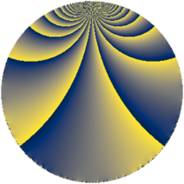# Properties

 Label 150.3.iLevel $150$ Weight $3$ Character orbit 150.i Rep. character $\chi_{150}(29,\cdot)$ Character field $\Q(\zeta_{10})$ Dimension $80$ Newform subspaces $1$ Sturm bound $90$ Trace bound $0$

# Related objects

## Defining parameters

 Level: $$N$$ $$=$$ $$150 = 2 \cdot 3 \cdot 5^{2}$$ Weight: $$k$$ $$=$$ $$3$$ Character orbit: $$[\chi]$$ $$=$$ 150.i (of order $$10$$ and degree $$4$$) Character conductor: $$\operatorname{cond}(\chi)$$ $$=$$ $$75$$ Character field: $$\Q(\zeta_{10})$$ Newform subspaces: $$1$$ Sturm bound: $$90$$ Trace bound: $$0$$

## Dimensions

The following table gives the dimensions of various subspaces of $$M_{3}(150, [\chi])$$.

Total New Old
Modular forms 256 80 176
Cusp forms 224 80 144
Eisenstein series 32 0 32

## Trace form

 $$80 q - 40 q^{4} + 20 q^{9} + O(q^{10})$$ $$80 q - 40 q^{4} + 20 q^{9} + 16 q^{10} + 20 q^{12} + 32 q^{15} - 80 q^{16} + 60 q^{19} - 60 q^{21} + 40 q^{22} + 116 q^{25} - 210 q^{27} - 40 q^{28} - 68 q^{30} + 180 q^{31} - 50 q^{33} - 120 q^{34} + 40 q^{36} - 40 q^{37} + 220 q^{39} + 32 q^{40} + 468 q^{45} + 120 q^{46} - 40 q^{48} - 680 q^{49} + 20 q^{51} - 120 q^{54} - 272 q^{55} - 156 q^{60} - 200 q^{61} - 830 q^{63} - 160 q^{64} + 160 q^{66} + 500 q^{67} - 280 q^{69} - 584 q^{70} + 120 q^{73} - 138 q^{75} - 80 q^{76} + 620 q^{78} + 400 q^{79} - 420 q^{81} + 180 q^{84} + 1632 q^{85} + 750 q^{87} + 160 q^{88} + 472 q^{90} - 340 q^{91} + 160 q^{94} + 20 q^{97} - 260 q^{99} + O(q^{100})$$

## Decomposition of $$S_{3}^{\mathrm{new}}(150, [\chi])$$ into newform subspaces

Label Dim $A$ Field CM Traces $q$-expansion
$a_{2}$ $a_{3}$ $a_{5}$ $a_{7}$
150.3.i.a $80$ $4.087$ None $$0$$ $$0$$ $$0$$ $$0$$

## Decomposition of $$S_{3}^{\mathrm{old}}(150, [\chi])$$ into lower level spaces

$$S_{3}^{\mathrm{old}}(150, [\chi]) \cong$$ $$S_{3}^{\mathrm{new}}(75, [\chi])$$$$^{\oplus 2}$$